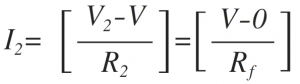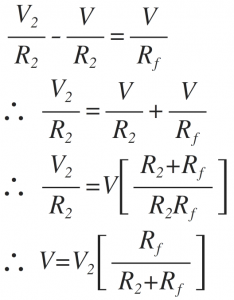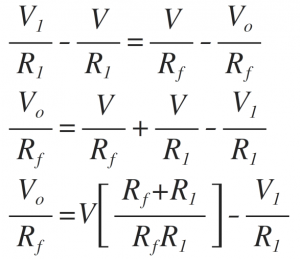# Subtractor/Difference Amplifier

The subtraction of the two input voltages is possible with the help of subtractor. The subtractor using op-amp is shown in figure below. It is also called as difference amplifier.The input signals applied are V1 and V2.
Let us assume that the non-inverting terminal is at potential 'V'. Due to virtual ground concept, the inverting terminal appears to be at the same potential 'V' as shown in the circuit diagram.

Let the current flowing through resistance R1 and R2 are I1 and I2 respectively. Since input current to the op-amp is zero, the two currents flows through the resistance Rf as shown in circuit diagram above. The current I2 is given asFrom the above equation voltage 'V' can be calculated asThe current I1 is given asSimplify the equation,Substituting the voltage 'V' from the equation we get,If R1=R2=RfThus at the output we get subtraction of the two input voltages.
The subtractor circuits are used to solve various mathematical equations.